# NCERT Class 10 Maths Triangles

In the introduction section of the chapter 6, it is mentioned that the aim is to study triangles that have the same shape but not the same size. Such triangles are known as similar triangles. Further, it is proposed that two polygons of the same number of sides are similar, if (i) their corresponding angles are equal and (ii) their corresponding sides are in the same ratio (or proportion). For the second exercise, the similarity of triangles is taken up and it is noted that two triangles are similar when - corresponding angles are equal and their corresponding sides are in the same ratio (or proportion). In the third part of the chapter, the criterion for similarity of triangles is presented (AAA, AA, SSS and SAS). Finally, the chapter covers concepts like the areas of similar triangles and the Pythagoras theorem.

## Chapter 6 Ex.6.1 Question 1

Fill in the blanks using the correct word given in brackets:

(i)   All circles are ___________. (congruent, similar)

(ii)  All squares are ___________. (similar, congruent)

(iii) All ___________ triangles are similar. (isosceles, equilateral)

(iv) Two polygons of the same number of sides are similar, if

(a) their corresponding angles are ____________ and

(b) their corresponding sides are ___________. (equal, proportional)

### Solution

(i) All circles are Similar. (congruent, similar)

Reasoning:

As we know that two similar figures have the same shape but not necessarily the same size. (Same size means radii of the circles are equal)

Steps:

Similar. Since the radii of all the circles are not equal.

(ii) All squares are Similar. (similar, congruent)

Reasoning:

As we know that two similar figures have the same shape but not necessarily the same size(same size means sides of the squares are equal.)

Steps:

Similar. Since the sides of the squares are not given equal.

(iii) All Equilateral. triangles are similar. (isosceles, equilateral)

Reasoning:

Anequilateral triangle has equal sides and equal angles.

Steps:

Equilateral. Each angle inan equilateral triangle is $${{60}^{{}^\circ }}$$.

(iv) Two polygons of the same number of sides are similar, if (a) their corresponding angles are Equal and (b) their corresponding sides are Proportional (equal, proportional).

Reasoning:

As we know that two polygons of same number of slides are similar if their corresponding angles are equal and all the corresponding sides are in the same ratio or proportion.

Steps:

(a) Since the polygons have same number of sides, we can find each angle using formula \begin{align}\left( {\frac{{2n - 4}}{n}} \right)\end{align} right angles. Here $$‘n’$$ is number of sides of a polygon.

(b) We can verify by comparing corresponding sides.

## Chapter 6 Ex.6.1 Question 2

Give two different examples of pair of

(i) similar figures

(ii) non-similar figures

### Solution

Steps(i):

(a) Two equilateral triangles of sides $$2$$ cm and $$6$$ cm.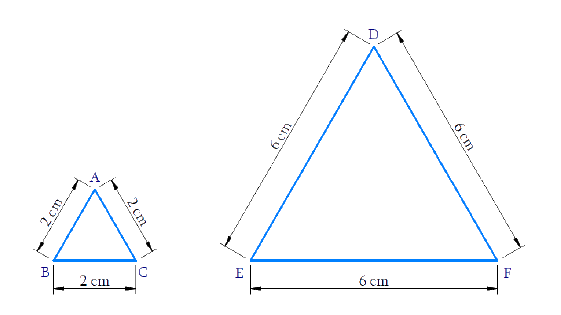$\Delta ABC\sim\Delta DEF\$$\sim\text{is similar to})$ (b) Two squares of sides \(3$$ cm and $$5$$ cm.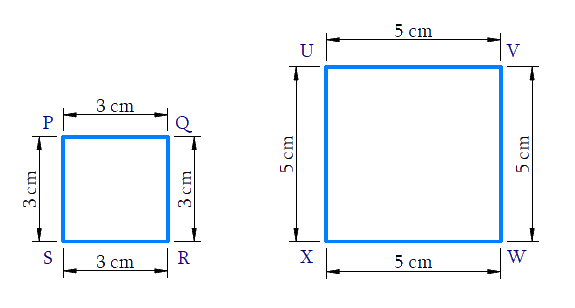$$\square PQRS\!\sim\!\square UVWX\\(\sim\text{is similar to})$$

Steps (ii) :

(a) A quadrilateral and a rectangle.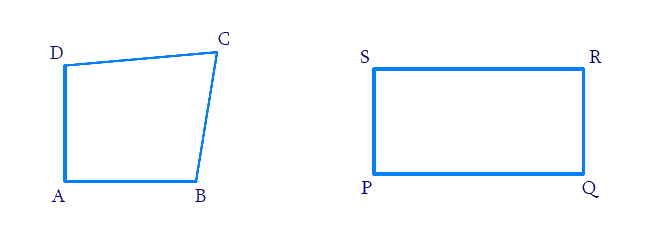(b) A triangle and a parallelogram.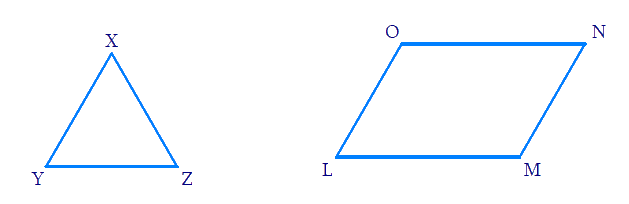## Chapter 6 Ex.6.1 Question 3

State whether the following quadrilaterals are similar or not: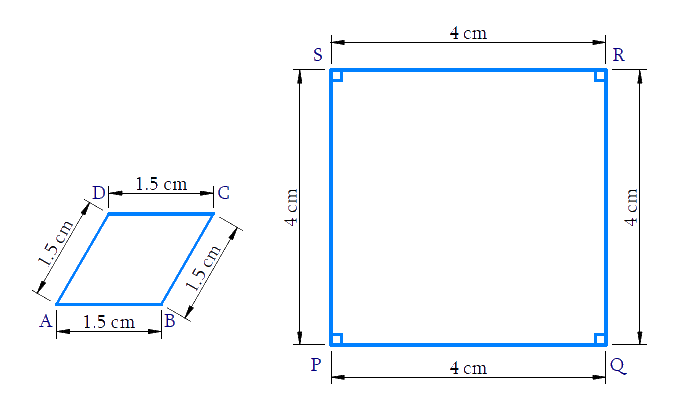### Solution

Reasoning:

Two polygons of the same number of sides are similar, if

(i) all the corresponding angles are equal and

(ii) all the corresponding sides are in the same ratio (or proportion).

Steps:

In Quadrilaterals $$ABCD$$ and $$PQRS$$

\begin{align}\frac{{AB}}{{PQ}} = \frac{{BC}}{{QR}} = \frac{{CD}}{{RS}} = \frac{{DA}}{{SP}} = \frac{{3}}{{8}}\end{align}

$$\Rightarrow$$ Corresponding sides are in proportion

But $$\angle A \ne \angle P;\angle B \ne \angle Q$$

$$\Rightarrow$$ Corresponding angles are not equal

$$\square ABCD \nsim \square PQRS$$

No, Quadrilateral $$ABCD$$ is not similar to Quadrilateral $$PQRS$$

Triangles | NCERT Solutions
Instant doubt clearing with Cuemath Advanced Math Program